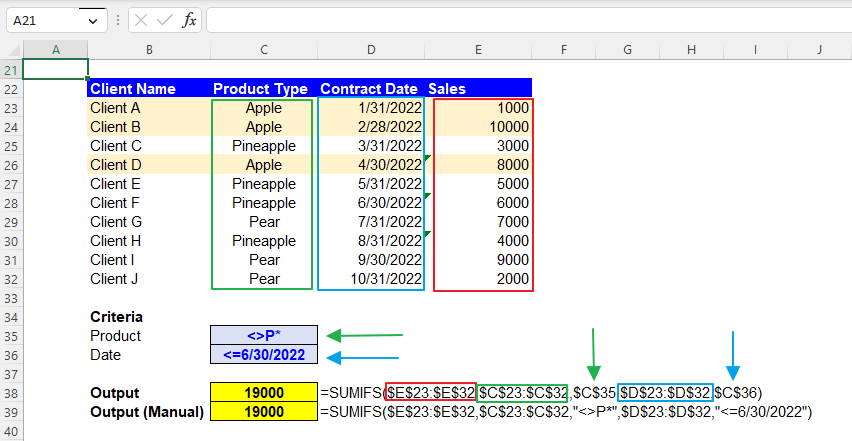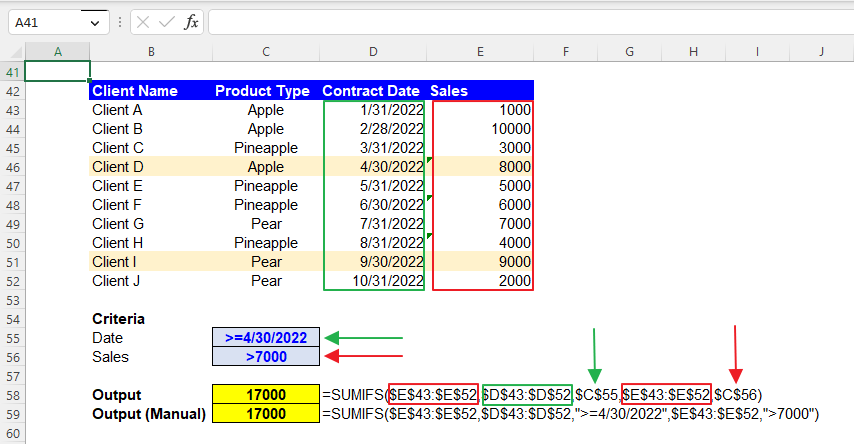Excel Formulas

# SUMIFS Function in Excel: Explained

In this article, you will learn how to use the SUMIFS formula in Excel.

## How does the SUMIFS function work in Excel?

The SUMIFS function in Excel allows you to sum the values in a specified range that meet multiple criteria.

## When do you use the SUMIFS formula in Excel?

The SUMIFS formula in Excel sums up the values in a selected range that meet multiple criteria. It is beneficial when you have a large dataset with multiple columns and want to sum a particular value based on certain conditions in the same or other columns.

For example, you might use the SUMIFS formula to:

1. Aggregate the sales for a specific product with a certain amount of sales.
2. Add up the volume of items sold by a particular salesperson within a specific date range.
3. Sum the hours worked by a specific employee in a certain department and pay period.

## How do I use the SUMIFS function in Excel?

The syntax for the SUMIFS function is as follows:

``=SUMIFS(sum_range, criteria_range1, criteria1, [criteria_range2, criteria2], ...)``

sum_range: The range of cells that you want to sum.

criteria_range1: The first range of cells that you want to evaluate using criteria1.

criteria1: The criterion in the form of a number, expression, or text that defines which cells in criteria_range1 will be added.

criteria_range2, criteria2…(optional): Additional ranges and their associated criteria.

Note 1: When you enter non-numeric criteria manually in the formula, remember that you enclose each text with double quotes.

Note 2: The SUMIFS formula is not case-sensitive.

Note 3: If the selected “sum_range” contains any “TRUE” or “FALSE”, the calculated result may be incorrect because the SUMIFS treats “TRUE” as 1 and “FALSE” as 0.

In the next section, you can see examples of the SUMIFS function with multiple conditions, such as numeric value and text.

## How to use the SUMIFS function with multiple criteria in Excel?

Before looking at some examples, learn basic operators and wildcards quickly.

If you can add a condition to a specific number of N, you can use the following logic operators.

>N: Greater than N

>=N: Greater than or equal to N

<N: Less than N

<=N: Less than or equal to N

=N: Equal to N

<>N: Not equal to N

Wildcards include three signs: an asterisk, a question mark, and a tilde, each of which has a different function.

”*” (Asterisk): Any character (e.g., “A*”: Any characters starting with A / “*B*”: Any characters containing B)

”?” (Question mark): The number of character(s) corresponds to the number of question mark(s). (e.g., ”????”: Any four characters)

”~” (Tilde): This negates the effect of the other wildcards next to the right and makes them work as literal marks without any function as wildcards. (e.g., ”*~?~?*”: Any characters containing two question marks)

Number and Text

Assume you have the dataset in the picture below and want to calculate the total sales from clients who recorded sales over 5000 and bought Pear. You can use the following SUMIFS formula:

sum_range: \$E\$3:\$E\$12 (Sales column)

criteria_range1: \$E\$3:\$E\$12 (Sales column)

criteria1: \$C\$15

criteria_range2: \$C\$3:\$C\$12 (Product Type column)

criteria2: \$C\$16

Although we recommend that you use cell references to input conditions as they give you more visibility and clarity of the criteria applied, if you can insert the criteria manually, you can create the formula below for this case.

``=SUMIFS(\$E\$3:\$E\$12,\$E\$3:\$E\$12,”>5000”,\$C\$3:\$C\$12,”Pear”)``

Text and Date

Next, imagine you have the same dataset and want to calculate the total sales from clients who purchased products whose names do not start with P on or before 6/30/2022. The formula should be as follows:

``=SUMIFS(\$E\$23:\$E\$32,\$C\$23:\$C\$32,\$C\$35,\$D\$23:\$D\$32,\$C\$36)``

sum_range: \$E\$23:\$E\$32 (Sales column)

criteria_range1: \$C\$23:\$C\$32 (Product Type column)

criteria1: \$C\$35

criteria_range2: \$D\$23:\$D\$32 (Contract Date column)

criteria2: \$C\$36

The following formula includes input criteria manually.

``=SUMIFS(\$E\$23:\$E\$32,\$C\$23:\$C\$32,”<>P*”,\$D\$23:\$D\$32,”<=6/30/2022”)``How to use the SUMIFS formula with text and date criteria in Excel

Date and Number

Lastly, assume that you have the same dataset and want to calculate the total sales from clients who recorded more than 7000 sales on or after 4/30/2022. You can use the formula below.

``=SUMIFS(\$E\$43:\$E\$52,\$D\$43:\$D\$52,\$C\$55,\$E\$43:\$E\$52,\$C\$56)``

sum_range: \$E\$43:\$E\$52 (Sales column)

criteria_range1: \$D\$43:\$D\$52 (Contract Date column)

criteria1: \$C\$55

criteria_range2: \$E\$43:\$E\$52 (Sales column)

criteria2: \$C\$56

If you want to generate the SUMIFS with manually input conditions, use the formula below.

``=SUMIFS(\$E\$43:\$E\$52,\$D\$43:\$D\$52,”>=4/30/2022”,\$E\$43:\$E\$52”>7000”)``How to use the SUMIFS function with date and number requirements

## Why do you use cell references in the SUMIFS formula in Excel?

As you have seen, you can input the “criteria” argument(s) manually. However, we recommend the cell reference approach (instead of manual inputs) because it gives you more visibility and clarity about the condition as the requirement can be seen in a cell and allows you to change the condition more quickly. You don’t need to put your cursor on the cell containing the formula or open the cell by hitting the F2 button to see in detail or revise the function. Note that you don’t need to enclose it with quotation marks when you put a criterion in a cell.

## What is the difference between the SUMIFS and SUMIF functions in Excel?

The SUMIFS and SUMIF functions in Excel are similar in that they both allow you to sum values in a specified range based on a certain criterion. The SUMIFS function, as you have learned, allows you to include multiple criteria to determine which cells should be added together. The SUMIF formula, on the other hand, only allows you to specify only one condition. Therefore, you should use the SUMIFS function when applying more than one standard to your dataset.

## Analyze your live financial data in a snap in Google Sheets

Are you learning this formula to visualize financial data, build a financial model, or conduct financial analysis? In that case, LiveFlow may help you automate manual workflows, update numbers in real-time, and save time. You can access various financial templates on our website, from the simple Income Statement to Multi-Currency Consolidated Financial Statement. Are you interested in this product but are an Excel user? That’s not a problem at all. You can connect Google Sheets to Excel quickly.